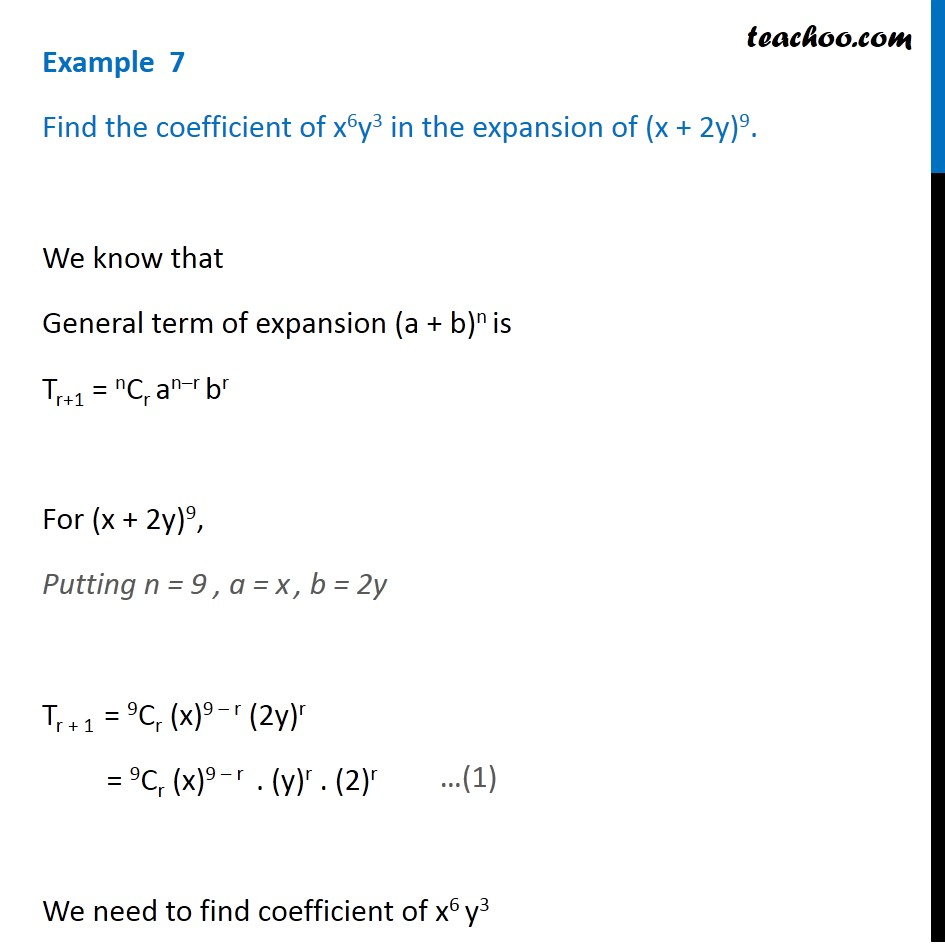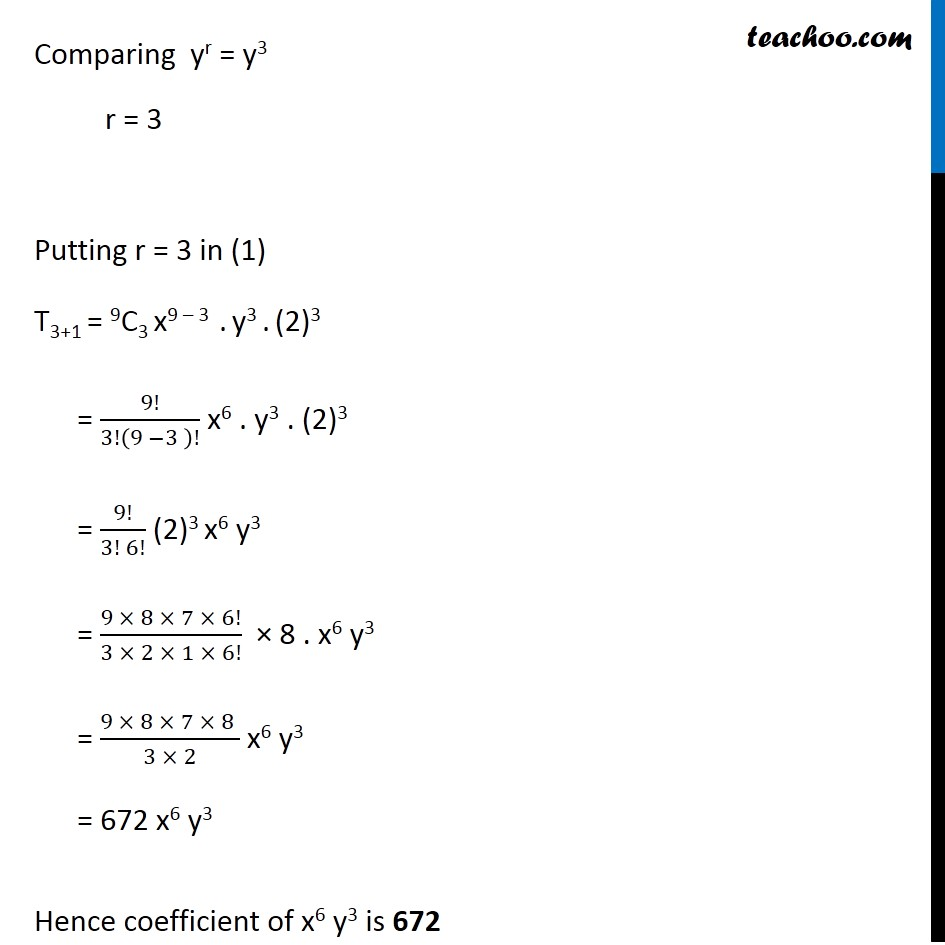Examples

Chapter 7 Class 11 Binomial Theorem
Serial order wiseLearn in your speed, with individual attention - Teachoo Maths 1-on-1 Class

### Transcript

Question 3 Find the coefficient of x6y3 in the expansion of (x + 2y)9. We know that General term of expansion (a + b)n is Tr+1 = nCr an–r br For (x + 2y)9, Putting n = 9 , a = x , b = 2y Tr + 1 = 9Cr (x)9 – r (2y)r = 9Cr (x)9 – r . (y)r . (2)r We need to find coefficient of x6 y3 Comparing yr = y3 r = 3 Putting r = 3 in (1) T3+1 = 9C3 x9 – 3 . y3 . (2)3 = 9!/3!(9 −3 )! x6 . y3 . (2)3 = 9!/(3! 6!) (2)3 x6 y3 = (9 × 8 × 7 × 6!)/(3 × 2 × 1 × 6!) × 8 . x6 y3 = (9 × 8 × 7 × 8 )/(3 × 2) x6 y3 = 672 x6 y3 Hence coefficient of x6 y3 is 672